#### Returning to Fourier analysis

Now consider the bottom part of Figure 11.12. The operation of a linear filter is easy to understand and compute in the frequency domain. This is the function obtained by performing the Fourier transform on the signal, which provides an amplitude for every combination of frequency and phase. This transform was briefly introduced in Section 11.1 and illustrated in Figure 11.3. Formally, it is defined for discrete-time systems as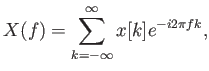(11.6)

in which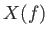is the resulting spectral distribution, which is a function of the frequency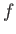. The exponent involves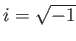and is related to sinusoids through Euler's formula: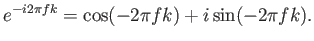(11.7)

Unit complex numbers are used as an algebraic trick to represent the phase. The inverse Fourier transform is similar in form and converts the spectral distribution back into the time domain. These calculations are quickly performed in practice by using the Fast Fourier Transform (FFT) [11,191].

Steven M LaValle 2020-11-11